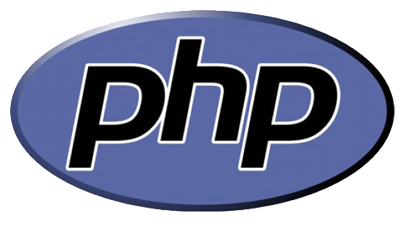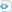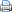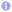# PHP – Converting bytes to a readable formatPosted by

The following function takes in a number of bytes and the number of decimal places required in the output and returns the result in a readable format:

 Code blockfunction readable_filesize(\$bytes, \$dp = 1) {
// Extensions used
\$extensions = array('B', 'KB', 'MB', 'GB', 'TB', 'PB');

foreach (\$extensions as \$ext) {
// If we can't divide again without getting < 1
if (\$bytes < 1024) {
return number_format(\$bytes, \$dp).\$ext;
}

\$bytes /= 1024;
}

// If we're here, we're at the end of the extensions array
// thus return what we have
return \$bytes.\$ext;
}

Use like this: